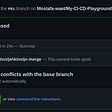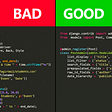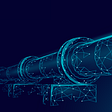# Adding Unfold and Fold Ops into OneFlow

Written by Zheng Zekang; Translated by Wang Kaiyan, Dong Wenwen

This article will cover the engineering of adding two ops that are often used when customizing convolution-related operations: Unfold and Fold. Read on!

# Starting From the Convolutional Layer

Convolution is a common but significant operation. Convolution in CNN is not the same thing as convolution in signal processing. CNN convolution is a two-dimensional cross-correlation operation. Chapter 5.1 of “Dive into Deep Learning” is taken as an example:

The elements in the window and the convolution kernel are multiplied and summed to get the output elements, a naive code is as follows (also from “Dive into Deep Learning”)

Here it is written with the help of the indexing feature of the numpy array. If you write it in C++, you will need more loop layers (which will be shown later). However, this is not a very efficient way of writing the loop computation, and it slows down the convolution operation significantly.

# First Sight of img2col

In order to improve the speed of the convolution operation, the img2col algorithm was invented, which essentially uses matrix multiplication to equate the convolution operation, with the following example diagram:

https://github.com/microsoft/AI-System/blob/main/docs/SystemforAI-4-Computer%20architecture%20for%20Matrix%20computation.pdf This the corresponding chapter of Microsoft’s AISystem repository, which is highly recommended!

You can see that img2col expands the input feature map further and then flattens the filter and does matrix multiplication of the two to get the same result.

# Understanding of img2col

Looking at the above figure, you may still not understand how this unfolds, so here we will elaborate on:

• Suppose the input feature map is (N, Cin, H, W), the convolution kernel parameters are (K, K, Cin, Cout), and the length and width of the output feature map are (Oh, Ow)

After img2col, the input feature map is transformed into a 3D vector `(N, Cin*K*K, Oh*Ow)`.

In addition the convolution kernel we will reshape into a two-dimensional vector `(Cout, K*K*Cin)`.

And these two vectors can be matrix multiplied and the output vector is `(N, Cout, Oh*Ow)`, which is the result we expect from the convolution.

The img2col algorithm is a space-for-time approach. After transforming the input, it obviously takes up more memory space, but the advantage is that the convolution operation can be done quickly with the help of matrix multiplication.

Then I will combine darknet’s native img2col and a blog to further explain it:

# Source Code of img2col

darknet’s img2col is actually ported from caffe. But here, for easy understanding, we take a simple CPU version as an example and do not consider the batch dimension.

To allow the reader to quickly run on the code, here I use a version of darknet img2col that I wrote as an example.

First we can determine the individual dimensions of the output tensor, where `out_h` and `out_w` are the height and width of the output, using the convolutional output formula:

`channel_cols` is what we mentioned before, and img2col will transform the second dimension to `C_in*K*K`.

Then it goes to the `channel_cols` times for loop

Then we need to get the corresponding input element based on the index of the output element currently being processed

The logic of how im_row is calculated is: the currently processed input element window start point: i.e. h*stride add the kh_offset offset within the window.

And the index is easier to calculate, because the output is (C, H, W), corresponding to the one-dimensional index that is

Finally, we take out the elements and assign them to the out array. Then we reshape the one-dimensional out array into the out_shape we derived earlier.

`img2col_get_pixel` is a function that legally takes elements, if there is an out-of-bounds range (for example, less than 0, or greater than Oh), then it is the part of the padding that is taken, at which point we return 0.

We can simply construct an array to verify the results (using the example from the Microsoft AI-System course as input)

The output is as expected:

col2img

col2img is the inverse of img2col, and interested readers can refer to the following blog:

https://blog.csdn.net/caicaiatnbu/article/details/102626135

A more complete illustration for understanding will be available later in the oneflow implementation section.

In DL frameworks, img2col and col2img have other names in Pytorch, namely Unfold and Fold. These two Ops are often used when you want to customize some convolution-related operations.

We assume that the input is a (1, 2, 4, 4) tensor, but inside the framework we usually store it as a one-dimensional array, as shown below:

However, we need the corresponding high-dimensional array index. OneFlow has an internal `NdIndexHelper` class, when constructing we can pass in the high-dimensional shape, and then call `OffsetToNdIndex` to perform the conversion from one-dimensional offset to high-dimensional index.

Here we construct a `NdIndexHelper` for the input and output Tensor respectively.

More specifically, we construct the output as a 6-dimensional form.

The next step is to derive which input element should be taken from the perspective of the output.

• The template parameter INDEX_T indicates the data type of the Index (can be int32_t, int64_t). NDIM indicates how many dimensions are being processed (here we have 2 dimensions). SDIM determines the location of the channel dimension, SDIM=1 is NHWC format, SDIM=2 is NCHW format (here we take 2).
• The input parameter index_a indicates the output NdIndexHelper, and index_b indicates the input NdIndexHelper.

From the previous analysis, we can see that the index of the two dimensions N, C is constant, so we can directly input

Then enter a loop with the number of NDIM==2

Here the index is calculated by deriving from the output to the input, and the formula is (taking H as an example):

If the input index calculated is less than 0 or greater than the width and height of the input, then it is to the padding, and we return true directly to indicate that it is out of bounds. If we can fetch the element, we assign this index to `index_b`, the input NdIndexHelper, and return false.

The decomposition operation is shown in the following figure:

The advantage of deriving from the output is that the whole operation is an elementwise operation, and we can do a loop with the number of elements of the output tensor to complete the whole unfold operation.

• First calculate the NdIndex of the currently processed output element based on offset
• Then determine the return value of the method UnfoldIndexTransform
• If it is false, then we can take the input element, convert its index to 1d offset and assign it to the output
• If it is true, then it is out of bounds and we can fill a previously set padding_value(0)

At this point, the entire img2col process has been completed, and the overall operation is shown in the following figure:

Fold is to fill each column back to the kxk

If you can understand Unfold, then the Fold here can also be easily understood. It’s just that the elements of the operation are reversed.

Following the previous index mapping logic, we enter a loop with the number of input elements, and compute the input NdIndex corresponding to the current offset.

If `FoldIndexTransform` returns false, compute the output offset and use atomic plus atomic add to accumulate input elements to that output position.

If you want to follow the code and reproduce the whole process, click the repo link to see the implementation in OneFlow : https://github.com/Oneflow-Inc/oneflow/pull/5675

# Conclusion

To be honest, we must say that this whole design is cunning and brilliant. Through the template, parameters can be expanded 1d, 2d, 3d, nchw, nhwc various formats, although it is not easy to understand intuitively.

The darknet version (same as Caffe) is a straightforward img2Col algorithm for beginners to get started with. You can use the two blogs above to understand the whole process.

Related articles：

Also, welcome to join our Discord group to discuss and ask OneFlow related questions, and connect with OneFlow contributors and users all around the world.

--

--

--

## More from OneFlow

OneFlow is a performance-centered and open-source deep learning framework.

Love podcasts or audiobooks? Learn on the go with our new app.

## How to Use Custom GitHub Checks Using Jenkins Pipeline?!## 7 Best Security Practices in Python Programming Language## 21 Techniques to Write Better Python Code## Swift for TensorFlow## Monads are not Rocket Surgery— Part Two: Result## Rails Generators: The Basics## OneFlow

OneFlow is a performance-centered and open-source deep learning framework.

## Lists of Articles Posted in 2021## How to run OpenVINO™ with Neural Compute Stick 2 on Linux## Inference in Production: 5 Factors that Impact It & the Hardware Usage Metrics to Track## Google’s New Patent Helps Drivers Keep Attention While Driving